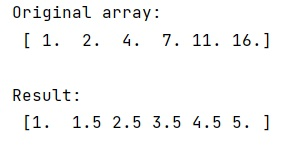Submitted by Pranit Sharma, on January 21, 2023

NumPy is an abbreviated form of Numerical Python. It is used for different types of scientific operations in python. Numpy is a vast library in python which is used for almost every kind of scientific or mathematical operation. It is itself an array which is a collection of various methods and functions for processing the arrays.

The numpy.gradient() method is used to find the gradient of an N-dimensional array. The gradient is computed using second-order accurate central differences in the interior points and either first or second-order accurate one-sides (forward or backward) differences at the boundaries. The returned gradient hence has the same shape as the input array.

Syntax:

```numpy.gradient(f, *varargs, axis=None, edge_order=1)
```

Parameter(s):

• f: array_like- An N-dimensional array containing samples of a scalar function.
• varargs: list of scalar or array, optional- Spacing between f values. Default unitary spacing for all dimensions. Spacing can be specified using:
• single scalar to specify a sample distance for all dimensions.
• N scalars to specify a constant sample distance for each dimension. i.e. dx, dy, dz, …
• N arrays to specify the coordinates of the values along each dimension of F. The length of the array must match the size of the corresponding dimension
• Any combination of N scalars/arrays with the meaning of 2. and 3.
• If axis is given, the number of varargs must equal the number of axes.
• edge_order: {1, 2}, optional- Gradient is calculated using N-th order accurate differences at the boundaries. Default: 1.

Let's understand with the help of an example,

## Python code to demonstrate the example of numpy.gradient() method

```# Import numpy
import numpy as np

# Creating a numpy array
arr = np.array([1, 2, 4, 7, 11, 16], dtype=float)

# Display original array
print("Original array:\n",arr,"\n")

# Display the result
print("Result:\n",res)
```

Output:What's New (MCQs)

Top Interview Coding Problems/Challenges!

IncludeHelp's Blogs

Languages: » C » C++ » C++ STL » Java » Data Structure » C#.Net » Android » Kotlin » SQL
Web Technologies: » PHP » Python » JavaScript » CSS » Ajax » Node.js » Web programming/HTML
Solved programs: » C » C++ » DS » Java » C#
Aptitude que. & ans.: » C » C++ » Java » DBMS
Interview que. & ans.: » C » Embedded C » Java » SEO » HR
CS Subjects: » CS Basics » O.S. » Networks » DBMS » Embedded Systems » Cloud Computing
» Machine learning » CS Organizations » Linux » DOS
More: » Articles » Puzzles » News/Updates